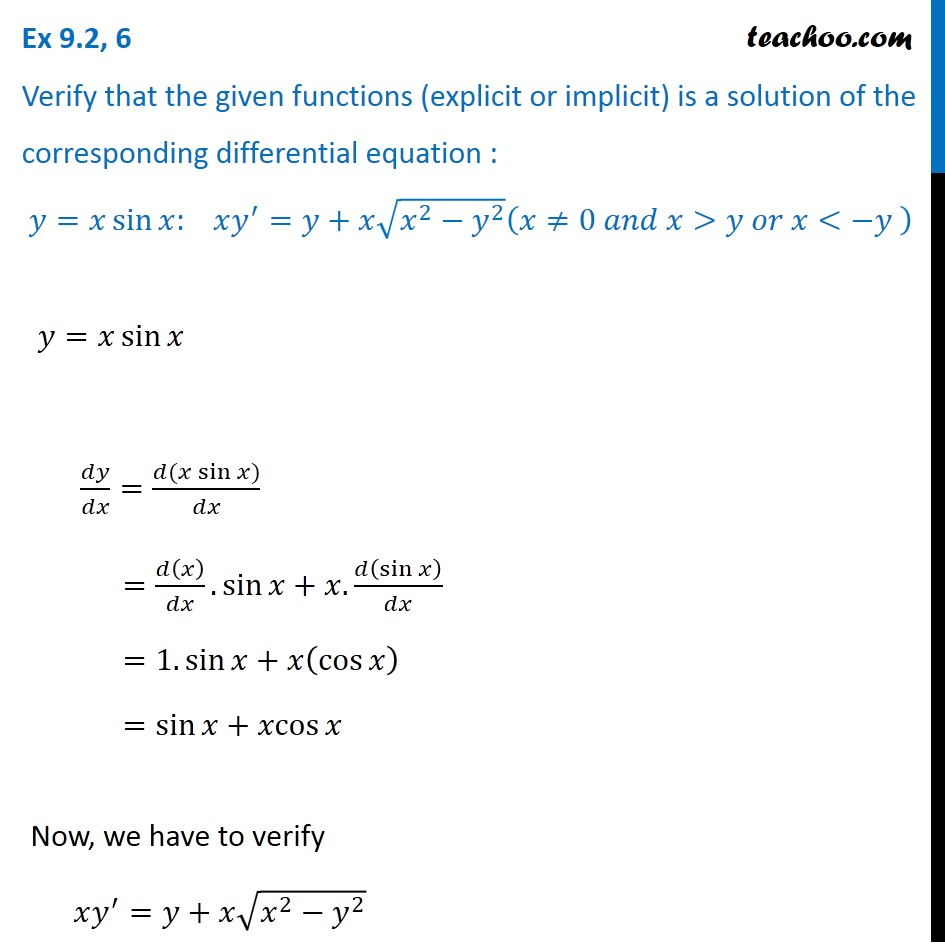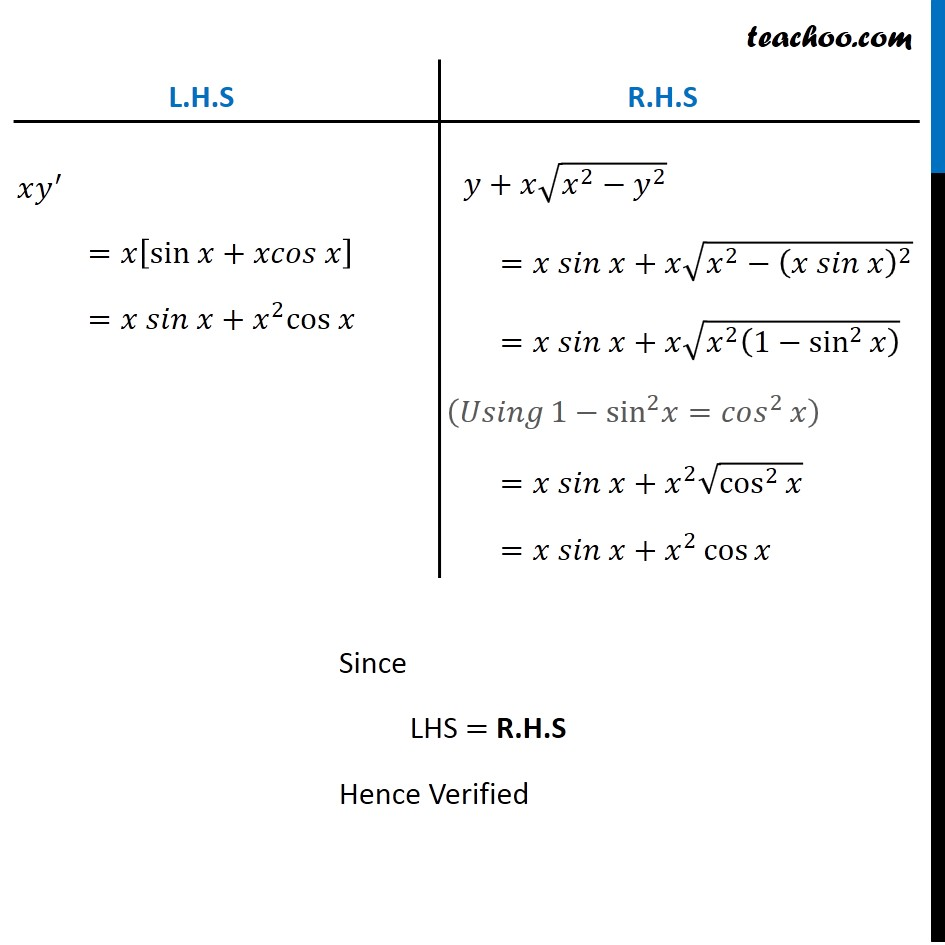Ex 9.2

Chapter 9 Class 12 Differential Equations
Serial order wiseLearn in your speed, with individual attention - Teachoo Maths 1-on-1 Class

### Transcript

Ex 9.2, 6 Verify that the given functions (explicit or implicit) is a solution of the corresponding differential equation : 𝑦=𝑥 sin⁡𝑥: 𝑥𝑦^′=𝑦+𝑥√(𝑥^2−𝑦^2 ) (𝑥≠0 𝑎𝑛𝑑 𝑥>𝑦 𝑜𝑟 𝑥<−𝑦 ) 𝑦=𝑥 sin⁡𝑥 𝑑𝑦/𝑑𝑥=(𝑑(𝑥 sin⁡𝑥))/𝑑𝑥 =𝑑(𝑥)/𝑑𝑥.sin⁡𝑥+𝑥.𝑑(sin⁡𝑥 )/𝑑𝑥 =1.sin⁡𝑥+𝑥(cos⁡𝑥 ) =sin⁡𝑥+𝑥cos⁡𝑥 Now, we have to verify 𝑥𝑦^′=𝑦+𝑥√(𝑥^2−𝑦^2 ) L.H.S 𝑥𝑦^′ =𝑥[sin 𝑥+𝑥𝑐𝑜𝑠 𝑥] =𝑥 𝑠𝑖𝑛 𝑥+𝑥^2 cos 𝑥 R.H.S 𝑦+𝑥√(𝑥^2−𝑦^2 ) =𝑥 𝑠𝑖𝑛 𝑥+𝑥√(𝑥^2−(𝑥 𝑠𝑖𝑛 𝑥)^2 ) =𝑥 𝑠𝑖𝑛 𝑥+𝑥√(𝑥^2 (1−sin^2⁡𝑥 ) ) =𝑥 𝑠𝑖𝑛 𝑥+𝑥^2 √(cos^2⁡𝑥 ) =𝑥 𝑠𝑖𝑛 𝑥+𝑥^2 cos⁡𝑥 Since LHS = R.H.S Hence Verified